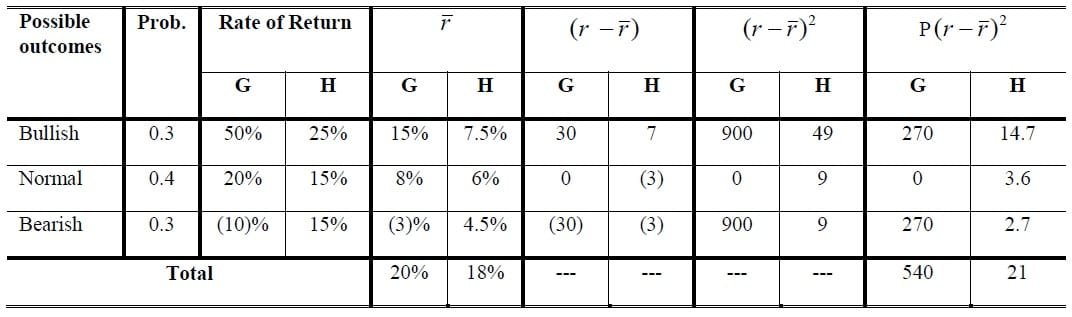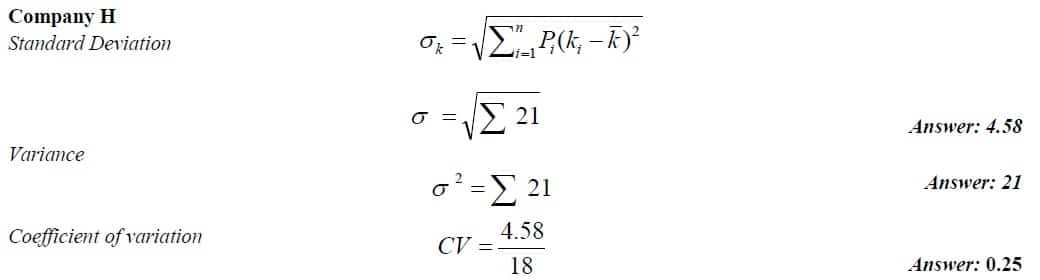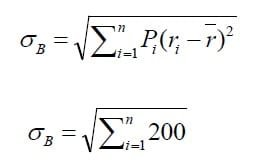Select Page

# Risk and Return Problems and Solutions

## Problem 1:

On the basis of expected Rate, Standard Deviation, Variance and Coefficient of variation decided which of the following company is best for investment (Single company Risk analysis).

### Solution:##On the basis of above mention risk indicators Company H is best.

Problem 2:

Following are the probability distribution of returns of portfolio of Stock A and Stock B in equal proportion of weight in each state of economy.  You are required to calculate Expected Return and Risk for individual Stocks?

##Problem 3:

If you deposit Rs. 1,000 in the bank at a nominal interest rate of 6 percent, you will have Rs. 1,060 at the end of the year. Suppose that the inflation rate during the year is also 6 percent. Find real amount in Rupees?

## Problem 4:

You save Rs. 100 and invest it at a nominal interest rate of 8%. Given the expected inflation is 5% per year, what is the real rate of return?

## Problem 5:

Suppose the current market value of Rs. 100 and no dividend income after one year. Find expected Rate of Return?

## Related Topics

Formula Sheet

Future Value and Present Value Tables

## Related Courses

Principles of Accounting

Cost Accounting

Principles of Finance